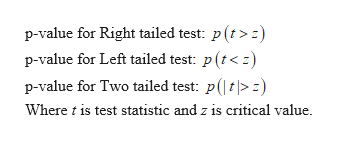Describe a hypothesis test study that would help your work or conclusions in some way. Describe what variable would be tested and what would be your guess of the value of that variable. Then include how the result, if the null were rejected or not, might change your conclusions or actions in some way. Use 1 scholarly reference.

Question

Describe a hypothesis test study that would help your work or conclusions in some way. Describe what variable would be tested and what would be your guess of the value of that variable. Then include how the result, if the null were rejected or not, might change your conclusions or actions in some way. Use 1 scholarly reference.

Step 1

Hypothesis test: A hypothesis test is a test to determine the significance of sample statistics corresponding to the population parameter at given level of significance.

Here, sample statistic would be tested and population parameter is the guess of the value of  variable.

Step 2

Decision rule: We obtain the value of test statistic from the given sample and  critical value from the respective distribut...help_outlineImage Transcriptionclosep-value for Right tailed test: p (t> p-value for Left tailed test: p (t<) p-value for Two tailed test: pt> Where is test statistic and z is critical value. fullscreen

Want to see the full answer?

See Solution

Want to see this answer and more?

Our solutions are written by experts, many with advanced degrees, and available 24/7

See Solution
Tagged in

Statistics